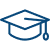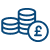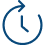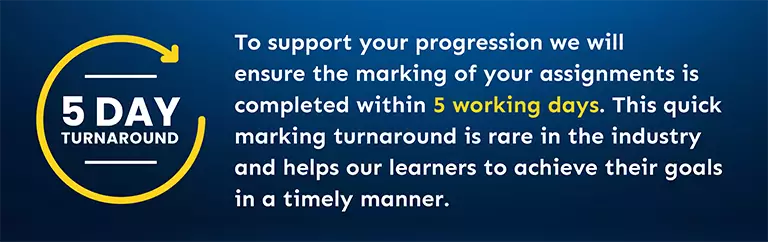[Image_Link]https://ct.pinterest.com/v3/?event=init&tid=2613995578594&pd[em]=&noscript=1 />## Tutor Support Included

Learning remotely doesn’t mean you’re on your own! You’ll be able to message your tutor through our industry-leading learning platform.## Flexible Payment Options

Enrol today from as little as £9.99. Buy Now, Pay no course fees until 1st September 2022!## Learn At Your Own Pace## Tutor Support Included

Learning remotely doesn’t mean you’re on your own! You’ll be able to message your tutor through our industry-leading learning platform.## Flexible Payment Options

Enrol today from as little as £9.99. Buy Now, Pay no course fees until 1st September 2022!# GCSE Mathematics - exams not included

100% online
learning
6 units
140 guided
learning hours

## This Course at a Glance

• Covers the entire Maths GCSE curriculum
• Learn how to use different mathematical techniques to solve problems
• Develop the ability to draw conclusions and reason mathematically
• Can be finished in as little as 6 months
• Covers both the Foundation and Higher tiers
• Complete your studies at home
• Exam not included

Not having Mathematical understanding can not only hinder your ability to progress in education, it can also prevent you from navigating and overcoming life’s daily challenges. As such, studying Maths at Level 2 can provide you with vital knowledge that will help you both academically and in your personal life.

Any learner looking to advance their Mathematical comprehension, be it to break down other challenging subjects and concepts, develop important problem-solving skills, or both, can do so online.

Our online GCSE Mathematics course covers the entire Level 2 Mathematics curriculum. So, you explore the modules at both the foundation and advanced level, to build your confidence and fluency in each one.

Not having Maths skills can become a barrier to entry for many of life's opportunities. This course aims to help you overcome these obstacles by taking you through the pillars of the subject by exploring algebra, ratio, geometry, probability, statistics, and many more.

This course will help you recognise the importance of Mathematics in life, and put to bed any fears you have surrounding the subject. On completion of the course, you will have developed strong knowledge, skills and understanding of Mathematical methods and concepts, and be able to solve problems without hesitation.

You can use this newfound confidence to tackle Mathematical related issues in work or your daily life. Or you can cement your qualification status by choosing to complete the GCSE Mathematics exam, which you will have to book and pay for separately.

## Getting Started

learndirect is the leading UK online course provider, with a broad range of approved GCSE courses that can provide learners with the Level 2 qualifications they need to apply for a job or move on to further education.

By studying this GCSE Mathematics course online, you are choosing to study the course content separately from the exam. This will give you the ability to develop your understanding of this vital subject at your own pace. Once enrolled, all the course materials you require are readily available in our online student portal.

The online nature of our courses appeals to learners who cannot or don’t want to learn within a classroom environment. Whether this is due to pre-existing commitments, or preferring home study over the distractions associated with group learning, choosing to learn online brings with it a host of benefits.

Simply log on and learn when it suits. Should you wish to complete your exam, you will need to book and pay for it separately. You can contact the learndirect team to organise this.

During your studies, you will benefit from the support of an experienced tutor. We also have flexible payment methods on offer that help make your education much more affordable.
OUR PASS RATE
GCSEs (National Average 82.6%)96%
Module 1: Number
1.1: Structure and Calculation
Upon completion of this unit, you will:
• Understand how to order positive and negative integers
• Understand decimals and fractions
• How to apply the four operations, including integers, decimals, proper and improper simple fractions, positive and negative mixed numbers
• Understand and use place value
• Know how to recognise and use relationships between operations, including inverse operations
• Be able to use conventional notation for priority of operations
• Be confident in using prime numbers, factors, multiples, common factors, common multiples, and more
• Be able to apply systemic listing strategies, including use of the product rule for counting
• Understand and recognise powers of 2,3,4,5, estimate powers and roots of any given positive number
• Know how to calculate with roots and with integer indices, and calculate with fractional indices
• Be able to calculate with fractions, calculate exactly with multiples of (π), calculate exactly with surds, simplify surd expressions involving squares and rationalise denominators
• Calculate with and interpret standard form
1.2: Fractions, Decimals, and Percentages
Upon completion of this unit, you will:
• Understand how to work with terminating decimals and corresponding fractions
• Be able to change recurring decimals into their corresponding fractions and vice versa
• Know how to identify and combine fractions and ratios
• Be confident in interpreting fractions and percentages as operators
1.3: Measures and Accuracy
Upon completion of this unit, you will:
• Understand how to use units of mass, time, length, money, and other measures using decimal quantities where appropriate
• Be able to estimate answers and check calculations using approximation and estimation
• Know how to accurately round numbers and measures
• Be able to use inequality notation to specify simple error intervals
• Understand how to apply and interpret limits of accuracy including upper and lower bounds
Module 2: Algebra
2.1: Notation, Vocabulary, and Manipulation
Upon completion of this unit, you will:
• Be able to use and interpret algebraic symbols
• Understand how to substitute numerical values into expressions and formulae
• Know how to use expressions, equations, inequalities, formulae, terms and factors, and include identities
• Be able to simplify and manipulate algebraic expressions, including those involving surds and algebraic fractions
• Understand mathematical formulae and how to rearrange formulae
• Know the difference between an identity and an equation
• Be able to argue mathematically to show algebraic expressions are equivalent, and use algebra to support and construct arguments, and include proofs
• Be able to interpret expressions as functions
• Be able to interpret the reverse process as the ‘inverse function’, and interpret the succession of two functions as a ‘composite function’
2.2: Graphs
Upon completion of this unit, you will:
• Know how to work with coordinates in all four quadrants
• Be able to plot graphs of equations that correspond to straight-line graphs
• Know how to find the equation of the line through two given points or through one point with a gradient
• Be able to use a specific form to identify perpendicular lines
• Understand how to identify and interpret gradients and intercepts of linear functions graphically and algebraically
• Know how to identify and understand roots, intercepts and turning points of quadratic functions graphically, deduce roots algebraically, and deduce turning points by completing the square
• Understand how to recognise, interpret, and draw graphs of linear functions and quadratic functions, including simple cubic functions and the reciprocal function, plus exponential functions for positive values, and the trigonometric functions (with arguments in degrees) for angles of any size
• Be able to sketch translations and reflections of a given function
• Be able to plot and interpret graphs to find solutions to problems, including reciprocal and  exponential graphs
• Be able to calculate or estimate gradients of graphs and areas under graphs (including quadratic and other non-linear graphs), and interpret results in cases such as distance-time graphs, velocity-time graphs and graphs in financial contexts
• Be able to recognise and use the equation of a circle with centre at the origin and find the equation of a tangent to a circle at a given point
2.3: Solving Equations and Inequalities
Upon completion of this unit, you will:
• Be confident in solving linear equations and finding solutions using a graph, including those with the unknown on both sides of the equation
• Know how to solve quadratic equations algebraically by factorising and find approximate solutions using a graph, including those that require rearrangement, plus completing the square and by using the quadratic formula
• Be able to solve two simultaneous equations in two variables, find approximate solutions using a graph, including linear/quadratic
• Know how to translate simple procedures into algebra, derive an equation (or two simultaneous equations), solve the equation(s) and interpret the solution
• Understand how to solve linear inequalities in one variable, represent the solution set on a number line, solve linear inequalities in one or two variable(s), and quadratic inequalities in one variable, and represent the solution set on a number line, using set notation and on a graph
2.4: Sequences
Upon completion of this unit, you will:
• Know how to generate terms of a sequence
• Be able to recognise and use sequences of triangular, cube and square numbers, including Fibonacci-type sequences, quadratic sequences, and simple geometric progressions, including other sequences, and those where surds are included
• Be able to deduce expressions to calculate the nth term of linear sequences, including quadratic sequences

Module 3: Ratio, Proportion and Rates of Change

Upon completion of this module, you will:

• Be confident in switching from time, length, area, volume, and mass to speed, prices, rates of pay in numerical contexts, plus compound units (eg density, pressure) in numerical and algebraic contexts
• Know how to use scale factors, maps, and scale diagrams
• Be able to express one quantity as a fraction of another
• Understand how to use ratio notation, including reduction
• Be able to divide and express a given quantity
• Know how to apply ratio to real contexts
• Be confident in expressing a multiplicative relationship between two quantities as a ratio or a fraction
• Understand and use proportion as equality of ratios
• Be able to relate ratios to fractions and to linear functions
• Know how to define and interpret percentages
• Understand how to work with percentages greater than 100% and solve problems involving percentages
• Be able to solve problems involving direct and inverse proportion
• Be able to use compound units such as speed, rates of pay and unit pricing, plus use compound units such as density and pressure
• Know how to use ratio notation to compare lengths, areas, volumes and scale factors, and make links to similarity (including trigonometric ratios)
• Be able to interpret equations that describe direct and inverse proportion, construct and interpret equations that describe direct and inverse proportion
• Be able to interpret the gradient of a straight-line graph as a rate of change, plus recognise and interpret graphs that illustrate direct and inverse proportion
• Be able to interpret the gradient at a point on a curve as the instantaneous rate of change, plus apply the concepts of average and instantaneous rate of change (gradients of chords and tangents) in numerical, algebraic and graphical contexts
• Be able to set up, solve and interpret the answers in growth and decay problems, including compound interest and work with general iterative processes
Module 4: Geometry and Measures
4.1: Properties and Constructions
Upon completion of this unit, you will:
• Understand points, lines, edges, parallel lines, right angles, polygons, and more
• Know how to use the standard conventions for labelling and making reference to the sides and angles of triangles
• Know how to draw diagrams based on written descriptions
• Be able to apply the properties of angles at a point, on a straight line, and vertically, use these to construct given figures and solve loci problems, and know that the perpendicular distance from a point to a line is the shortest distance to the line
• Understand alternative and corresponding angles on parallel lines
• Be able to use the basic congruence criteria for triangles
• Be confident in applying special types of quadrilaterals, triangles, and other figures
• Be able to identify, describe, and construct congruent and similar shapes, including fractional and negative scale factors
• Be able to apply angle facts, triangle congruence, similarity and properties of quadrilaterals to conjecture and derive results about angles and sides, including Pythagoras’ theorem and the fact that the base angles of an isosceles triangle are equal, and use known results to obtain simple proofs
• Be able to describe the changes and invariance achieved by combinations of rotations, reflections and translations
• Understand how to identify and apply circle definitions and properties, including: tangent, arc, sector and segment
• Be able to apply and prove the standard circle theorems concerning angles, radii, tangents and chords, and use them to prove related results
• Know how to solve geometrical problems on coordinate aces
• Be able to identify the faces, surfaces, edges and vertices of cubes, cuboids, cylinders, pyramids, prisms, and more
• Know how to interpret plans and elevations of 3D shapes, plus construct and interpret plans and elevations of 3D shapes
4.2: Mensuration and Calculation
Upon completion of this unit, you will:
• Know how to use standard units of measure and related concepts
• Understand how to measure line segments and angles in geometric figures, including interpreting maps and scale drawings and use of bearings
• Be able to apply formulae to calculate area of triangles, parallelograms, and trapezia; volume of cuboids and other right prisms (including cylinders)
• Understand the formulae: circumference of a circle, area of a circle, how to calculate perimeters of 2D shapes, including circles, areas of circles and composite shapes, plus the surface area and volume of spheres, pyramids, cones and composite solids
• Be able to calculate arc lengths, angles and areas of sectors of circles
• Be able to apply the concepts of congruence and similarity, including the relationships between lengths in similar figures, including the relationships between lengths, areas and volumes in similar figures
• Understand the formulae for: Pythagoras’ theorem and the trigonometric ratios, and be able to apply them to find angles and lengths in right-angled triangles in two dimensional figures, plus apply them to find angles and lengths in right-angled triangles and, where possible, general triangles in two and three dimensional figures
• Understand the exact values and apply the rules of sine, cosine and more, to find unknown lengths and angles
• Be able to calculate the area, sides or angles of any triangle
4.3: Vectors
Upon completion of this unit, you will:
• Understand how to describe translations as 2D vectors
• Be able to apply addition and subtraction of vectors, multiplication of vectors by a scalar, and diagrammatic and column representations of vectors, plus use vectors to construct geometric arguments and proofs
Module 5: Probability
Upon completion of this module, you will:
• Understand how to record, describe, and analyse probability outcomes using tables and frequency trees
• Know how to apply ideas of randomness, fairness, and likely events to calculate expected outcomes of experiments
• Be able to relate expected frequencies to theoretical probability
• Understand how to apply the property that the probabilities of an exhaustive set of outcomes sum to 1, and apply the property that the probabilities of an exhaustive set of mutually exclusive events sum to 1
• Understand that empirical unbiased samples tend towards theoretical probability distributions, with increasing sample size
• Be able to enumerate sets and combinations of sets systematically, using tables, grids and Venn diagrams, including using tree diagrams
• Know how to construct theoretical possibility spaces for single and combined experiments with equally likely outcomes and use these to calculate theoretical probabilities
• Be able to calculate the probability of independent and dependent combined events, including using tree diagrams and other representations, and know the underlying assumptions
• Be able to calculate and interpret conditional probabilities through representation using expected frequencies with two-way tables, tree diagrams and Venn diagrams
Module 6: Statistics
Upon completion of this module, you will:
• Be able to infer properties of populations or distributions from a sample, whilst knowing the limitations of sampling
• Understand how to interpret and construct tables, charts, and diagrams, including tables and line graphs for time series data
• Be able to construct and interpret diagrams for grouped discrete data and continuous data, ie histograms with equal and unequal class intervals and cumulative frequency graphs, and know their appropriate use
• Know how to interpret, analyse, and compare the distributions of data sets from univariate empirical distributions, including box plots, quartiles and inter-quartile range
• Be able to use statistics to describe a population
• Understand how to use and interpret scatter graphs of bivariate data, recognise correlation, know that it does not indicate causation, draw estimated lines of best fit, make predictions, interpolate and extrapolate apparent trends whilst knowing the dangers of so doing

Learners will be pleased to know there are no entry requirements to enrol on this course. All you need to get started is a computer/laptop and a stable internet connection!

*You will have access to the course for 12 months. You can purchase course extensions and/or the exams hereAs you make your way through your online GCSE Maths course, you will be assessed via Tutor Marked Assignments (TMAs) to keep you on track, and receive email support throughout.

*Please note, there is no exam included in this course bundle. Should you wish to take the exam to achieve your official GCSE grade and qualification, you will have to book this separately through learndirect. Alternatively, we offer a GCSE Maths course bundle that includes this exam.

### Pearson Edexcel

If you decide to take the GCSE exam at the end of this course and pass, you will have gained a GCSE in Mathematics. This will enable you to progress to further and higher education programmes.

Pearson Edexcel are the UK's largest awarding body and we are regulated by Ofqual (England), SQA Accreditation (Scotland), CCEA Accreditation (Northern Ireland) and Qualifications Wales (Wales). They offer academic and vocational qualifications that are globally recognised and benchmarked, with educational excellence rooted in names like Edexcel, BTEC, EDI and LCCI.## Career

A GCSE in Mathematics allows you to progress to A Level study or apply for an entry-level job role, should they only require GCSEs as part of their applications. A GCSE Mathematics qualification will also allow you to enrol on an RQF course, or an Access to Higher Education Diploma, which will subsequently enable you to study a degree in a related subject.